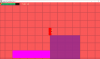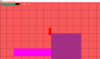# SOLVEDProblems with collisions at the edge (2D platformer)

#### IgnacioG

##### Member
Hi everyone, I need help with a somehow specific problem.

I'm working in a 2D pixel platformer and obviously one of the first things that I implemented was collisions with platforms. To be honest collisions aren't my forte so when I found this problem I ignore it at first but is been a couple of months and now I need a solution for it.

This problem is that the player get stuck in solid platforms when he falls to close to the edge, this can happen in two situations:
Jumping and falling above the platform very close to it's edge.
Going to the edge of the platform, running to one side to fall and running to the other side as soon as you start falling. This method was used for the pictures below (The red square draws the collision mask of the player).When the player get stucked you can't move horizontally and sometimes you can jump out of it depending on how deep into the platform is the player, but you have to move horizontally in the air after jumping or you get stucked again.

This is the code for collisions

GML:
`````` #region Horizontal Collision
if(round(hSPD) != 0)
{

if(place_meeting(x+round(hSPD),y,obj_solid))
{
repeat(abs(round(hSPD)))
{
if(!place_meeting(x+sign(hSPD),y,obj_solid))
{
x += sign(hSPD);
}
else
{

break;
}
}
hSPD = 0;
}
}

x += round(hSPD);

#endregion
#region Vertical Collision
if(round(vSPD) != 0)
{
if(place_meeting(x,y+round(vSPD),obj_solid))
{
repeat(abs(round(vSPD)))
{
if(!place_meeting(x,y+sign(vSPD),obj_solid))
{

y += sign(vSPD);

}
else
{
break;
}
}
vSPD = 0;
}

}
y += round(vSPD);
#endregion``````

other important stuff about the game:

I'm working with small sprites and because of that the camera's proportions are 426x240
vSPD and hSPD are rounded because gravity and acceleration works with decimals. If I don't round this values the player's sprite starts to have a wave-like movement if there's a change is it's position.
This also happens with one way platforms, but the player just falls form it all together.

Any sort of help would be appreciated#### Bulltron

##### Member
Try it like this:

vSPD = round (vSPD);

if (place_meeting(x,y+vSPD,obj_solid))
{
while(!place_meeting(x,y+sign(vSPD),obj_solid))
{
y += sign(vSPD);
}
vSPD = 0;
}

y += vSPD;

#### Ecoo

##### Member
u need to chack both too like:
GML:
``````hSPD = round(hSPD);
vSPD = round(vSPD);

if(place_meeting(x + hSPD, y, obj_solid)){
while(!place_meeting(x + sign(vSPD), y, obj_solid)){
x += sign(hSPD);
}
hSPD = 0;
}
if(place_meeting(x, y + vSPD, obj_solid)){
while(!place_meeting(x , y + sign(vSPD), obj_solid)){
y += sign(vSPD);
}
vSPD = 0;
}
if(place_meeting(x+hSPD, y+vSPD, obj_solid)){
while(!place_meeting(x + sign(vSPD), y, obj_solid)){
x += sign(hSPD);
}
hSPD = 0;
vSPD = 0;
}
x += hSPD;
y += vSPD;``````
the 3rd "if" ask for diagonal rais or fall and go only on the horizontal axis to the wall.

#### IgnacioG

##### Member
Try it like this:

vSPD = round (vSPD);

if (place_meeting(x,y+vSPD,obj_solid))
{
while(!place_meeting(x,y+sign(vSPD),obj_solid))
{
y += sign(vSPD);
}
vSPD = 0;
}

y += vSPD;
I tried this when I started to program the player's collision. But it didn't worked :/ .

#### IgnacioG

##### Member
u need to chack both too like:
GML:
``````hSPD = round(hSPD);
vSPD = round(vSPD);

if(place_meeting(x + hSPD, y, obj_solid)){
while(!place_meeting(x + sign(vSPD), y, obj_solid)){
x += sign(hSPD);
}
hSPD = 0;
}
if(place_meeting(x, y + vSPD, obj_solid)){
while(!place_meeting(x , y + sign(vSPD), obj_solid)){
y += sign(vSPD);
}
vSPD = 0;
}
if(place_meeting(x+hSPD, y+vSPD, obj_solid)){
while(!place_meeting(x + sign(vSPD), y, obj_solid)){
x += sign(hSPD);
}
hSPD = 0;
vSPD = 0;
}
x += hSPD;
y += vSPD;``````
the 3rd "if" ask for diagonal rais or fall and go only on the horizontal axis to the wall.
I implemented the third if. It does detect the collision in diagonal, but the clipping keeps happening. Am I missing something else?.
Also now sometimes when the player gets stuck it gets teleported way off the original position in an instant.

#### Ecoo

##### Member
I implemented the third if. It does detect the collision in diagonal, but the clipping keeps happening. Am I missing something else?.
Also now sometimes when the player gets stuck it gets teleported way off the original position in an instant.
sry my mistake. i fergot to check the second speed and reversed it too. in the 3rd "if" is this the correct "while"
GML:
``````while(!place_meeting(x + sign(hSPD), y + sign(hSPD), obj_solid)){
x += sign(hSPD);
}``````
or u make the 3rd "if" like this. maybe it is then some smother movement, because he has his constant sdp on the x axis.
GML:
``````if(place_meeting(x+hSPD, y+vSPD, obj_solid)){
vSPD = 0;
}``````
and in the 1st "if u need to check `while(!place_meeting(x + sign(hSPD), y, obj_solid)){` hSpd not vSpd...
here the whole code again.

GML:
``````hSPD = round(hSPD);
vSPD = round(vSPD);

if(place_meeting(x + hSPD, y, obj_solid)){
while(!place_meeting(x + sign(hSPD), y, obj_solid)){
x += sign(hSPD);
}
hSPD = 0;
}
if(place_meeting(x, y + vSPD, obj_solid)){
while(!place_meeting(x , y + sign(vSPD), obj_solid)){
y += sign(vSPD);
}
vSPD = 0;
}
if(place_meeting(x + hSPD, y + vSPD, obj_solid)){
vSPD = 0;
}
x += hSPD;
y += vSPD;``````

#### IgnacioG

##### Member
sry my mistake. i fergot to check the second speed and reversed it too. in the 3rd "if" is this the correct "while"
GML:
``````while(!place_meeting(x + sign(hSPD), y + sign(hSPD), obj_solid)){
x += sign(hSPD);
}``````
or u make the 3rd "if" like this. maybe it is then some smother movement, because he has his constant sdp on the x axis.
GML:
``````if(place_meeting(x+hSPD, y+vSPD, obj_solid)){
vSPD = 0;
}``````
and in the 1st "if u need to check `while(!place_meeting(x + sign(hSPD), y, obj_solid)){` hSpd not vSpd...
here the whole code again.

GML:
``````hSPD = round(hSPD);
vSPD = round(vSPD);

if(place_meeting(x + hSPD, y, obj_solid)){
while(!place_meeting(x + sign(hSPD), y, obj_solid)){
x += sign(hSPD);
}
hSPD = 0;
}
if(place_meeting(x, y + vSPD, obj_solid)){
while(!place_meeting(x , y + sign(vSPD), obj_solid)){
y += sign(vSPD);
}
vSPD = 0;
}
if(place_meeting(x + hSPD, y + vSPD, obj_solid)){
vSPD = 0;
}
x += hSPD;
y += vSPD;``````

Thanks, I also had to stop the horizontal speed in the third "if", but now the collisions work.